Courses

# Graph Theory (Basic Level)

## 10 Questions MCQ Test Question Bank for GATE Computer Science Engineering | Graph Theory (Basic Level)

Description
This mock test of Graph Theory (Basic Level) for GATE helps you for every GATE entrance exam. This contains 10 Multiple Choice Questions for GATE Graph Theory (Basic Level) (mcq) to study with solutions a complete question bank. The solved questions answers in this Graph Theory (Basic Level) quiz give you a good mix of easy questions and tough questions. GATE students definitely take this Graph Theory (Basic Level) exercise for a better result in the exam. You can find other Graph Theory (Basic Level) extra questions, long questions & short questions for GATE on EduRev as well by searching above.
QUESTION: 1

### A non-planar graph with minimum number of vertices has

Solution:

K5 is smallest non-planar graph in terms of number of vertices.
The number of vertices in K5 is 5 and number of edges in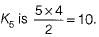QUESTION: 2

### Maximum number of edges in a n-node undirected graph without self loops is

Solution:

The graph containing maximum number of edges in a n-node undirected graph without self loops is complete graph.
The number of edges in complete graph with n-node,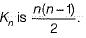QUESTION: 3

### The minimum number of colours required to colour the following graph, such that no two adjacent vertices are assigned the same colour, is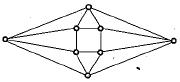Solution:

An assignment of the colors 1,2,3 and 4 to the vertices of the graph is shown below such that the graph is properly colored.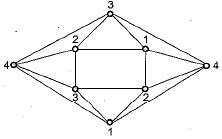So 4 colours are required.
[Note: The graph is a planar graph. The four colour theorem says that the chromatic number of a planar graph is at most = 4]

QUESTION: 4

What is the maximum number of edges in an acyclic undirected graph with n vertices?

Solution:

Acyclic graph are graphs which does not contain cycle.
For maximum number of edges each vertex connect to other vertex only if it does not from a cycle i.e. from a tree with n vertices, which has maximum 77-1 edges. Ex. with 4 vertices, 3 edges maximum.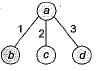QUESTION: 5

Which one of the following graphs is NOT planner?

Solution:

G1 is same as K3 3 which is known to be non planar G2, G3 and G4 can be redrawn as follows so that they are planar.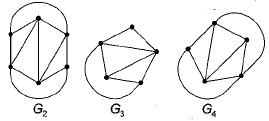QUESTION: 6

Let G be the non-planar graph with the minimum possible number of edges. Then G has

Solution:

K5 and K3, 3 are the smallest non planar graphs. K5 has 5 vertices and 5C2 = 10 edges and K3, 3 has 6 vertices and 3 x 3 = 9 edges . So, the non planar graph with minimum number of edges is K3, 3 with 9 edges and 6 vertices.
[Note: K5 is the non planar graph with minimum number of vertices]

QUESTION: 7

Which one of the following is TRUE for any simple connected undirected graph with more than 2 vertices?

Solution:

In a simple connected undirected graph (with more than two vertices), at least 2 vertices must have same degree, since if this is not true, then all vertices would have different degrees, A graph with all vertices having different degrees is not possible to construct (can be proved as a corollary to the Havell-Hakimi theorem). Notice that it is possible to construct graphs satisfying choices a, c and d.

QUESTION: 8

In a binary tree with n nodes, every node has an odd number of descendants. Every node is considered to be its own descendant. What is the number of nodes in the tree that have exactly one child?

Solution:

A tree with 1 node is not possible, since it is given that every node has exactly 1 child.
Now consider a tree with 2 nodes (a is the root)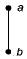Now a has exactly one child. Number of descendents of a = 2. But this contradicts the given fact that every node has an odd number of descendents.
Now consider atree with 3 nodes. Since, every- node has exactly one child, it must be of the form,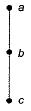Here a has 3 descendents, b has 2 descendents and c has one. Again we have contradiction in that b does not have odd number of descendents. Similarly can show that for tree with 4, 5, 6 ... nodes, it is not possible to have all nodes with odd number of descendents! So correct answer is the tree has 0 nodes, i.e., choice (a).

QUESTION: 9

The degree sequence of a simple graph is the sequence of the degrees of the nodes in the graph in decreasing order. Which of the following sequences can not be the degree sequence of any graph?​
I. 7, 6, 5, 4, 4, 3, 2, 1
lI. 6, 6,6, 6, 3, 3, 2, 2
III. 7, 6, 6, 4, 4, 3, 2, 2
IV. 8, 7, 7, 6, 4,2, 1,1

Solution:

Havell-Hakimi algorithm can be used to check whether a given degree sequence is a graph or not.
The algorithm is
1. Remove top node of the sequence.
2. Subtract "1" from as m any nodes in remaining sequence as the degree of top node that was removed.
3. Rearrange this sequence in non increasing order.
4. Check if resulting sequence is a graph.
5. Proceed again to step 1.
If the given sequence is not a graph we will see a violation in step 4, such as presence of negative degrees in the sequence. Otherwise the algorithm will bottom out with a degree sequence consisting of only even number of 1 ’s and any number of 0’s.
Now applying the algorithm to the degree sequences I, II, III and IV, one by one: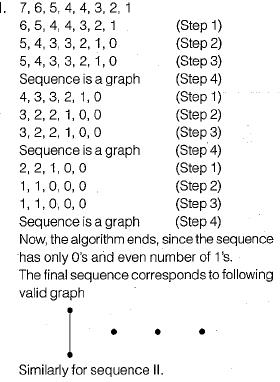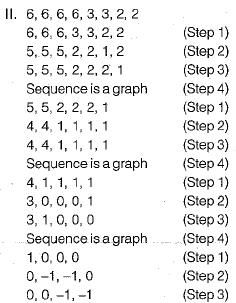The sequence is not a graph (Step 4), since negative degrees not possible in a valid graph. So, algorithm ends.
II is cannot be the degree sequence of any graph. Similarly we can show that III is degree sequence of some graph and IV is not a degree sequence of any graph.

QUESTION: 10

K4 and Q3 are graphs with the following structure: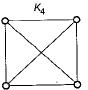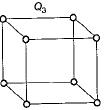Which one of the following statements is TRUE in relation to these graphs?

Solution:

The planar embedding of K4 and Q3 is shown below: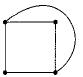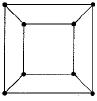So both graphs are planar.